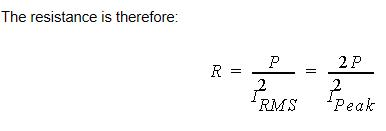## Electronics

#### Mismatch in Resistance from impedance matrix and resistance calculated from ohmic loss

•vignesh
Subscriber

Hello,

I used eddy current solver to obtain ac resistance of a high frequency transformer through impedance matrix. It is mentioned in maxwell help that the resistance is calculated from ohmic loss. Then calculated ohmic loss with the help of field calculator which matches the  result in solid loss. so there is no issue in calculated ohmic loss and then calculated the resistance from ohmic loss. But the obtained resistance is not matching the resistance displayed by Impedance matrix. Resistance is calculated using the formula below,Resistance from matrix- 781mohm

Resistance calculated from ohmic loss- 237.8mohm.

The difference looks huge.

I performed above exercise because during some design changes to reduce loss, I found about 37% reduction in ohmic loss but reduction in resistance was only about 6 % for same current magnitude.

I repeated the same for another high frequency device (inductor), here too I found mismatch.

This created a suspicion that the mistake is in my side or with solver ?

Used Ansys 18.0 for above simulations.

Hope someone can help me.

Thanks

Vignesh